# C++ Equality Operators

In C++ programs sometimes we need to check for equality of two variables or two expressions. The result of which is a boolean value such as true or false. The equality operators help us compare two numbers or expressions.

This will change the flow of the program in different directions. There are two equality operators:

The equality operators are similar to relational operators, but they are not part of relational operators. The working of equality operator is to check the equality and nothing else.

The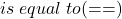operator is confusing for some people because it resembles the assignment operator. They are not the same. The equality operatorcannot be used to assign a value, this will result in a compilation error.

## Example Program: Is equal to (==)

#include <cstdlib>
#include <iostream>
using namespace std;
int main()
{
//Variable declaration

int a, b, c;

//Variable initialization

a = 200;
b = 300;
c = 200;

//Expression with eqaulity operator

if( a == c)
{
cout << "A is equal to C" << "\n";
}
else
{
cout << "A is not equal to C"<< "\n";
}
system("PAUSE");
return EXIT_SUCCESS;
}

## Output:

A is equal to C

In the above example, the result of the equality expression is true.

## Example Program: Is not equal to (!=)

The is not equal to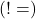can also output two Boolean value –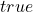or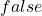depending on the expression.

#include <cstdlib>
#include <iostream>
using namespace std;
int main()
{
//Variable declaration

int a, b, c;

//Variable initialization

a = 200;
b = 300;
c = 200;

//Expression with eqaulity operator

if( a != b)
{
cout << "A is not equal to B" << "\n";
}
else
{
cout << "A is equal to B"<< "\n";
}
system("PAUSE");
return EXIT_SUCCESS;
}

## Output:

A is not equal to B

Now, we have modified the previous program slightly and if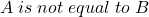meaning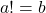is true statement, then the output of equality expression in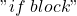will be executed.

This will print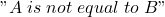.